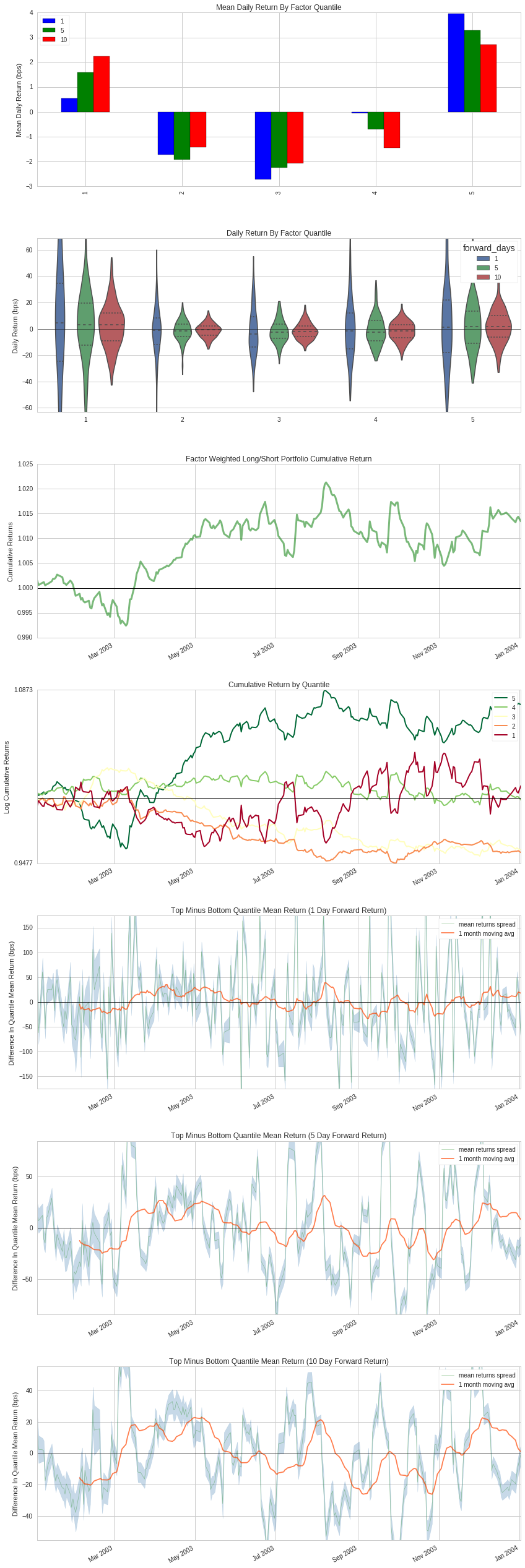Notebook

# Alphalens Example Tear Sheet¶

Alphalens is designed to aid in the analysis of "alpha factors," data transformations that are used to predict future price movements of financial instruments. Alpha factors take the form of a single value for each asset on each day. The dimension of these values is not necessarily important. We evaluate an alpha factor by considering daily factor values relative to one another.

It is important to note the difference between an alpha factor and a trading algorithm. A trading algorithm uses an alpha factor, or combination of alpha factors to generate trades. Trading algorithms cover execution and risk constraints: the business of turning predictions into profits. Alpha factors, on the other hand, are focused soley on making predictions. This difference in scope lends itself to a difference in the methodologies used to evaluate alpha factors and trading algorithms. Alphalens does not contain analyses of things like transaction costs, capacity, or portfolio construction. Those interested in more implementation specific analyses are encouaged to check out pyfolio (https://github.com/quantopian/pyfolio), a library specifically geared towards the evaluation of trading algorithms.

In :
import numpy as np
import pandas as pd
import alphalens
from quantopian.research import run_pipeline
from quantopian.pipeline import Pipeline
from quantopian.pipeline.data.builtin import USEquityPricing
from quantopian.pipeline.data import morningstar
from quantopian.pipeline.factors import CustomFactor, Returns, AverageDollarVolume
from quantopian.pipeline.classifiers.morningstar import Sector

MORNINGSTAR_SECTOR_CODES = {
-1: 'Misc',
101: 'Basic Materials',
102: 'Consumer Cyclical',
103: 'Financial Services',
104: 'Real Estate',
205: 'Consumer Defensive',
206: 'Healthcare',
207: 'Utilities',
308: 'Communication Services',
309: 'Energy',
310: 'Industrials',
311: 'Technology' ,
}

In :
class MomentumRanking(CustomFactor):
inputs=[USEquityPricing.close]
window_length=252

def compute(self, today, assets, out, close):
value_table = pd.DataFrame(index=assets)
value_table['mom1'] = close[-1] / close[-20] - 1
value_table['mom2'] = close[-1] / close[-60] - 1
value_table['mom3'] = close[-1] / close[-125] - 1
value_table['mom4'] = close[-1] / close - 1

out[:] = value_table.rank(ascending = False).mean(axis=1)

class market_cap(CustomFactor):
inputs = [morningstar.valuation.market_cap]
window_length = 1

def compute(self, today, assets, out, cap):
out[:] = cap[-1]

class efficiency_ratio(CustomFactor):
inputs = [USEquityPricing.close, USEquityPricing.high, USEquityPricing.low]
window_length = 252

def compute(self, today, assets, out, close, high, low):
lb = self.window_length
e_r = np.zeros(len(assets), dtype=np.float64)
a=np.array([high[1:(lb):1]-low[1:(lb):1],
abs(high[1:(lb):1]-close[0:(lb-1):1]),
abs(low[1:(lb):1]-close[0:(lb-1):1])])
b=a.T.max(axis=1)
c=b.sum(axis=1)
e_r=abs(close[-1]-close) / c
out[:] = e_r

In :
# Make the Pipeline
mkt_screen = market_cap()
mkt_cap = mkt_screen.top(3000)
er = efficiency_ratio()
er_filter = er > 0.0
volume_filter = AverageDollarVolume(window_length=20) > 0.5e6
monthly_returns_filter = Returns(window_length=20) > 0

total_filter = (mkt_cap & er_filter & volume_filter & monthly_returns_filter)
combo_rank = MomentumRanking()

pipe = Pipeline(
columns={
'Momentum':combo_rank,
'Sector': Sector()
},
screen = total_filter
)

In :
# Run the Pipeline
start_date = '2003-01-01'
end_date = '2004-01-01'
results = run_pipeline(pipe, start_date, end_date)
results = results.fillna(value=0.)

In :
# Get Pricing Data
assets = results.index.levels.unique()
# We need to get a little more pricing data than the
# length of our factor so we can compare forward returns.
# We'll tack on another month in this example.
pricing = get_pricing(assets, start_date, '2004-02-01', fields='open_price')

In :
momentum_factor = results['Momentum']
sectors = results['Sector']

alphalens.tears.create_factor_tear_sheet(momentum_factor,
pricing,
sectors=sectors,
sector_names=MORNINGSTAR_SECTOR_CODES,
days=(1, 5, 10))

Returns Analysis

1 5 10
Ann. alpha 0.032 0.020 0.010
beta -0.048 -0.049 -0.030
Mean Daily Return Bottom Quantile (bps) 0.535 1.591 2.231
Mean Daily Spread (bps) 2.395 -0.321 -0.652
Information Analysis

1 5 10
IC Mean 0.010 0.009 0.010
IC Std. 0.117 0.152 0.140
t-stat(IC) 1.392 0.945 1.163
p-value(IC) 0.165 0.346 0.246
IC Skew 0.380 0.226 -0.100
IC Kurtosis 0.187 -0.330 -0.103
Ann. IR 1.389 0.943 1.161
Turnover Analysis

Top Quantile Bottom Quantile
Mean Turnover 0.287 0.139
Mean Factor Rank Autocorrelation 0.972
<matplotlib.figure.Figure at 0x7f1fc4f47390>